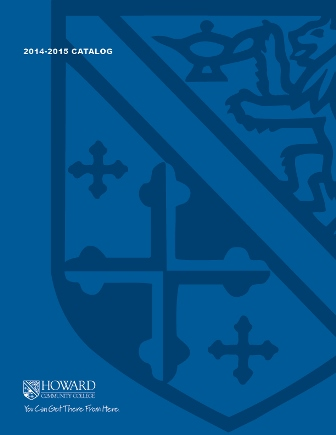2017-2018 College Catalog# MATH 127 Concepts of Mathematics I

This course is for students in the elementary education and early childhood education programs. Students will study the structural aspects of mathematics and the 'why' of arithmetical computations. Mental arithmetic is a required component of this course. Topics include sets, functions, logic, numeration systems, algorithms and their historical development, estimation, mental computations, and elementary number theory. Special emphasis is given throughout the course to problem-solving techniques. MATH 127 is not a mathematics core course.

4

### Prerequisite

C or better in MATH 070 or appropriate score on mathematics placement test

4 hours weekly

### Course Objectives

1. 1. Solve a variety of problems using Polya's problem solving techniques.
2. 2. Explain the steps of common arithmetic algorithms
3. 3. Compute in non-decimal number systems.
4. 4. Perform mental calculations and estimations.
5. 5. Occasionally use a calculator to help solve mathematical problems.
6. 6. State examples and proofs from number theory.
7. 7. Apply rules of logic to mathematical and other problems.
8. 8. Use mathematical symbols correctly.

### Course Objectives

1. Solve a variety of problems using Polya's problem solving techniques.

2. Explain the steps of common arithmetic algorithms

3. Compute in non-decimal number systems.

4. Perform mental calculations and estimations.

5. Occasionally use a calculator to help solve mathematical problems.

6. State examples and proofs from number theory.

7. Apply rules of logic to mathematical and other problems.

8. Use mathematical symbols correctly.